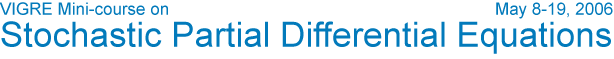David Nualart's Abstract Speakers Schedule & Notes Participants Visitor Information Contact Home Title: An introduction to Malliavin Calculus and its applications to SPDEs Abstract: The aim of these lectures is to first introduce the stochastic calculus of variations (Malliavin Calculus) for a Gaussian process and to establish the basic criteria for the existence and smoothness of densities. We will present two types of applications of Malliavin Calculus to SPDEs: Absolute continuity and smoothness of the density for the law of the solution at a fixed time of the stochastic heat and wave equations, assuming a nondegeneracy condition on the diffusion coefficient. Existence and uniqueness of a solution for the one-dimensional stochastic heat equation driven by a space-time white noise, when the diffusion coefficient is bounded away from zero and the drift is irregular (regularization effect). Prerequisites: Good working knowledge of Ito stochastic calculus and basic properties of Gaussian processes. Some basic properties of the stochastic heat and wave equations. VIGRE       U of U Math